search
Search
Publish
Guest 0reps
Thanks for the thanks!
close
chevron_left Matplotlib
Cancel
Post
account_circle
Profile
exit_to_app
Sign out
search
keyboard_voice
close
Searching Tips
Search for a recipe: "Creating a table in MySQL"
Search for an API documentation: "@append"
Search for code: "!dataframe"
Apply a tag filter: "#python"
Useful Shortcuts
/ to open search panel
Esc to close search panel
to navigate between search results
d to clear all current filters
Enter to expand content previewDoc SearchCode Search BetaSORRY NOTHING FOUND!
mic
Start speaking...Voice search is only supported in Safari and Chrome.
Shrink
Navigate to
A
A
share
thumb_up_alt
bookmark
arrow_backShareTwitterFacebook
chevron_left Matplotlib
thumb_up
2
thumb_down
0
chat_bubble_outline
0
auto_stories new
settings

# Getting Started with Matplotlib

Programming
chevron_right
Python
chevron_right
Matplotlib
schedule Jun 9, 2022
Last updated
local_offer PythonMatplotlib
Tags

# What is Matplotlib?

Matplotlib is a data visualization library in Python. Within the library, the `pyplot` module is commonly used for creating graphs and figures. Here are some reasons that make Matplotlib great:

• it allows you to develop professional plots using minimal lines of code

• highly customizable and lots of control over line styles, colors and font etc.

• performant even when working with large arrays as it can be used together with NumPy

# Importing Matplotlib

To import the `matplotlib.pyplot` module:

``` import matplotlib.pyplot as plt ```

Note that, by convention, we always use the alias `plt` for the `pyplot` module.

# Terminology

First it is important to understand the components of a figure in Matplotlib. The components are hierarchical:

• `Figure` object: the whole figure

• `Axes` object: belongs to a `Figure` object and is the space where we add the data to visualize

• `Axis` object: belongs to an `Axes` object. `Axis` object can be categorized as `XAxis` or `YAxis`.

The following figure illustrates the hierarchical relationship between the three objects: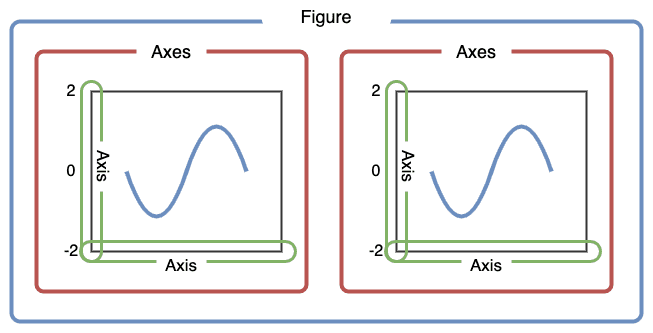The following figure also shows some of the more detailed elements composing a figure in Matplotlib: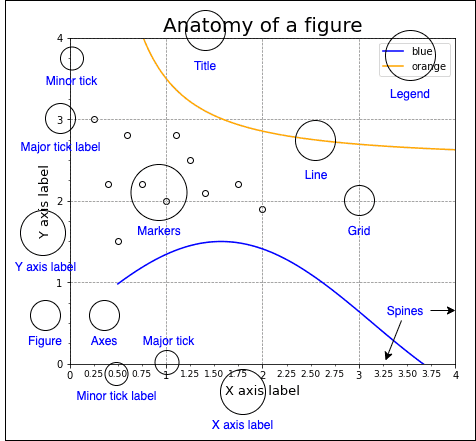# Approaches to plotting

There are two main approaches to plotting in Matplotlib:

• Object-Oriented Interface

• Pyplot Interface

NOTE

If you need granular control over how a graph is to be plotted, then the Object-Oriented approach is recommended as it provides more flexibility and control.

## Object-Oriented Interface

In this approach, we create `Figure` objects that will hold `Axes` objects (i.e. plots). We do this explicitly, and this in turn allows for a greater level of control and customization when constructing our figures.

The following code snippet demonstrates how to plot a line graph using the object-oriented interface:

``` import matplotlib.pyplot as plt# Initialize a Figure objectfig = plt.figure()# Add an Axes object to the Figure using add_subplot(1,1,1)# (1,1,1) here tells to add a 1x1 grid, 1st subplotax = fig.add_subplot(1,1,1)x = [1,2,3]y = [1,2,3]# Plot the data and show itax.plot(x, y)plt.show() ```

This renders the following graph: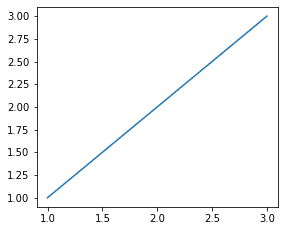Instead of generating the `Figure` and `Axes` object separately, we can initialize them together:

``` fig, ax = plt.subplots()ax.plot(x, y)plt.show() ```

## Pyplot Interface

The Pyplot interface will automatically generate and set the `Figure` / `Axes` objects you work with for you. This means you will continue to layer on changes to the same `Figure` using `pyplot` commands. This is different to the Object-Oriented interface where we explicitly specify which `Figure` or `Axes` object to apply our changes to.

WARNING

Although the Pyplot interface is a convenient and fast way to plot simple graphs, it isn't suited for making more complicated plots such as subplots.

Using the Pyplot interface, we are able to easily create simple figures. For example, to create a basic line chart:

``` x = [1,2,3]y = [1,2,3]plt.plot(x,y)plt.show() ```

This generates the following plot: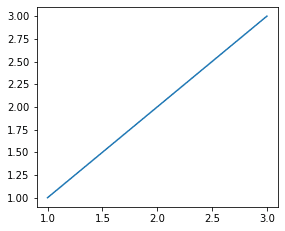In this case, the `plt.plot(~)` call is implicitly creating a `Figure` instance and an `Axes` instance in the background.

# Basics

## Drawing a bar chart

To draw a bar chart using the Pyplot interface:

``` plt.bar(["Blue", "Red"], [2,5])plt.show() ```

To perform the same using the object-oriented approach:

``` fig, ax = plt.subplots()ax.bar(["Blue", "Red"], [2,5])plt.show() ```

Both pieces of code result in a bar chart like below: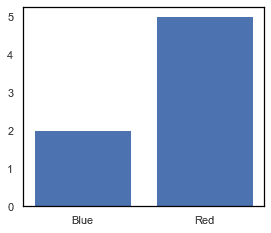## Drawing a histogram

To draw a histogram showing the distribution of some numbers using the Pyplot interface:

``` nums = [1,1,2,3,3,3,3,3,4,5,6,6,6,7,8,8,9,10,12,12,12,12,14,18]_ = plt.hist(nums)_ = plt.xlabel('Number')_ = plt.ylabel('Frequency')plt.show() ```

To perform the same using the object-oriented approach:

``` nums = [1,1,2,3,3,3,3,3,4,5,6,6,6,7,8,8,9,10,12,12,12,12,14,18]fig,ax = plt.subplots()ax.hist(nums)ax.set_xlabel('Number')ax.set_ylabel('Frequency')plt.show() ```

Both pieces of code result in a histogram as follows: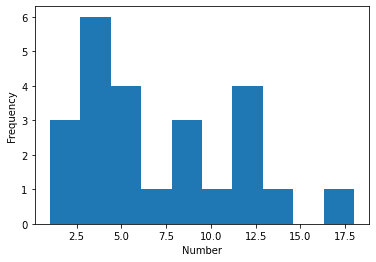## Drawing a scatter plot

To draw a basic 2D scatter plot using the Pyplot interface:

``` plt.scatter([5,2,3], [1,2,4])plt.show() ```

To draw the same 2D scatter plot using the object-oriented interface:

``` fig, ax = plt.subplots()ax.scatter([5,2,3], [1,2,4])plt.show() ```

Both pieces of code result in a scatter plot like the below: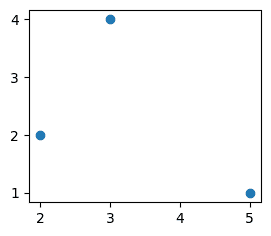To add a title to a plot using Pyplot interface:

``` plt.plot([1,2])plt.title("My Graph")plt.show() ```

To perform the same using the object-oriented interface:

``` fig, ax = plt.subplots()ax.plot([1,2])ax.set_title("My Graph")plt.show() ```

Both pieces of code produce the following plot: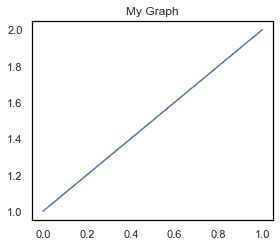To add axis labels using the Pyplot interface:

``` plt.plot([1,2])plt.xlabel("X Axis")plt.ylabel("Y Axis")plt.show() ```

To perform the same using the object-oriented interface:

``` fig, ax = plt.subplots()ax.plot([1,2])ax.set_xlabel("X Axis")ax.set_ylabel("Y Axis")plt.show() ```

Both pieces of code produce the following plot with labeled X and Y Axis: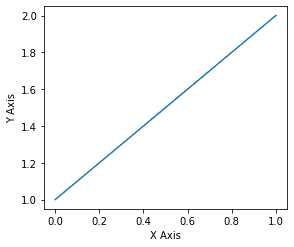To add a legend using the Pyplot interface:

``` plt.plot([1,2,3], label="blue", color="blue") # add the label parameter hereplt.plot([3,2,1], label="red", color="red") # add the label parameter hereplt.legend() # to embed the legendplt.show() ```

To perform the same using the object-oriented interface:

``` fig, ax = plt.subplots()ax.plot([1,2,3], label="blue", color="blue")ax.plot([3,2,1], label="red", color="red")ax.legend()plt.show() ```

Both pieces of code produce the following: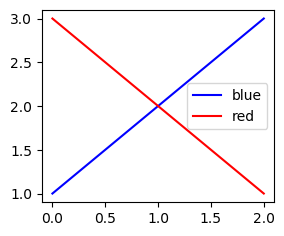To show grid lines using the Pyplot interface use the `plot.grid(~)` method:

``` plt.plot([1,2,3])plt.grid(color="gray", linestyle="--", linewidth=0.7)plt.show() ```

To perform the same using the object oriented interface:

``` fig, ax = plt.subplots()ax.plot([1,2,3])ax.grid(color="gray", linestyle="--", linewidth=0.7)plt.show() ```

Both pieces of code produce the following: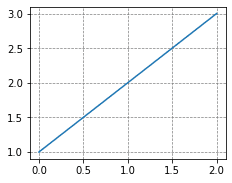## Saving figures

To save a figure in Matplotlib as a png file:

``` plt.plot([1,2]) # Your graphplt.savefig("my_curve.png") ```

This will save a file named "my_curve.png" in the same directory as your Python script.

To save a figure in Matplotlib as a pdf file:

``` plt.plot([1,2]) # Your graphplt.savefig("my_curve.pdf") ```

This will save a file named "my_curve.pdf" in the same directory as your Python script.